# RESULTS (The choices of electron pair geometry and molecular shape are linear, bent, trigonal planar, trigonal...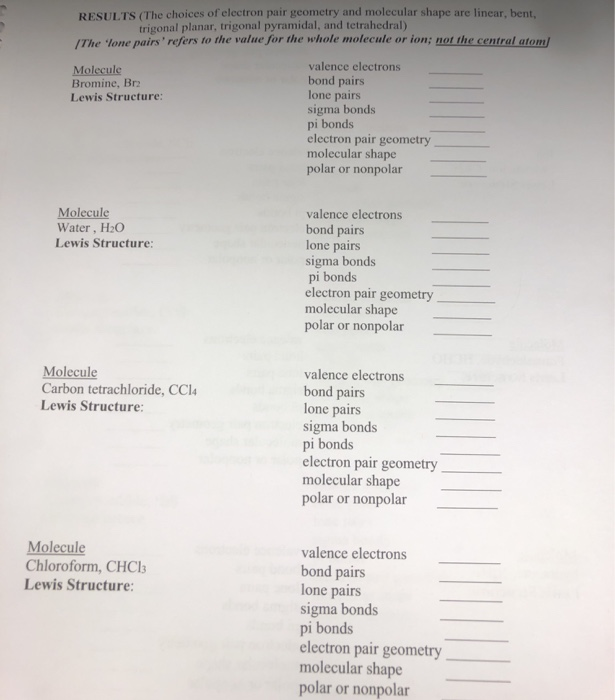RESULTS (The choices of electron pair geometry and molecular shape are linear, bent, trigonal planar, trigonal pyramidal, and tetrahedral) /The lone pairs' refers to the value for the whole molecule or ion; not the central atom/ valence electrons Molecule Bromine, Br bond pairs lone pairs sigma bonds pi bonds electron pair geometry molecular shape polar or nonpolar Lewis Structure: Molecule Water, H2O Lewis Structure: valence electrons bond pairs lone pairs sigma bonds pi bonds electron pair geometry molecular shape polar or nonpolar Molecule Carbon tetrachloride, CCl Lewis Structure: valence electrons bond pairs lone pairs sigma bonds pi bonds electron pair geometry molecular shape polar or nonpolar Molecule Chloroform, CHCla Lewis Structure: valence electrons bond pairs lone pairs sigma bonds pi bonds electron pair geometry molecular shape polar or nonpolar

Answer : Molecule Br2 : Lewis Structure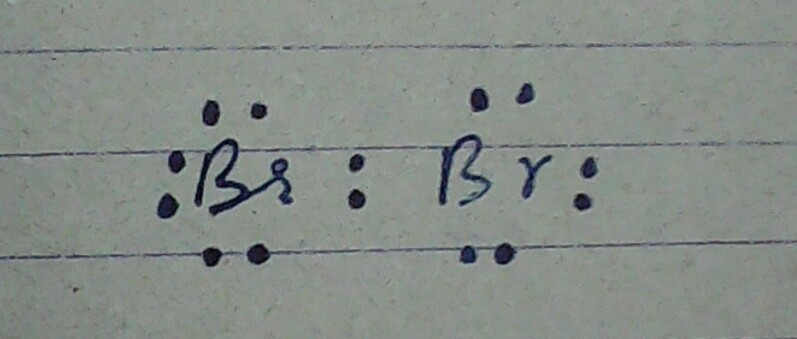Valance electrons = 14

Bond pairs = 1

Lone pairs = 6

Sigma bonds = 1

Pi-bonds = 0

Electron pair geometry = tetrahedral**** with respect to both 'Br' atoms

Molecular shape = Linear

Polar or non-polar : Br2 is a non- polar molecule because it is symmetrical.

----------------------

Molecule : H2O Lewis Structure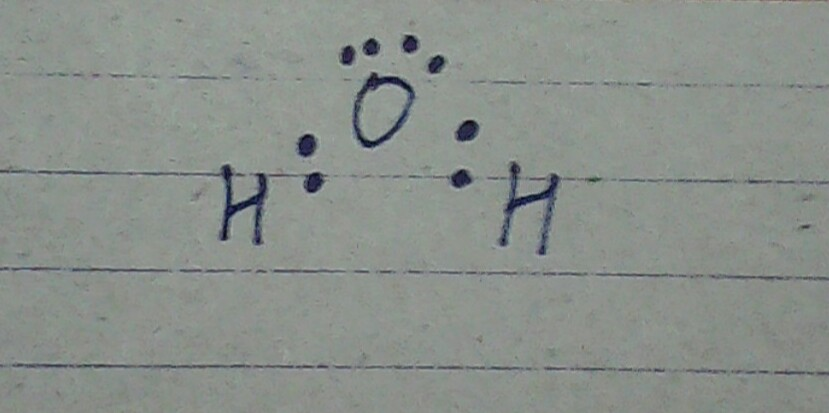Valance electrons : 8

Bond pairs = 2

Lone pairs = 2

Sigma bonds= 2

Pi-bond = 0

Electron pair geometry = Tetrahedral

Molecular shape = Bent shape

H2O is a polar molecule.

-------------------

Molecule: CCl4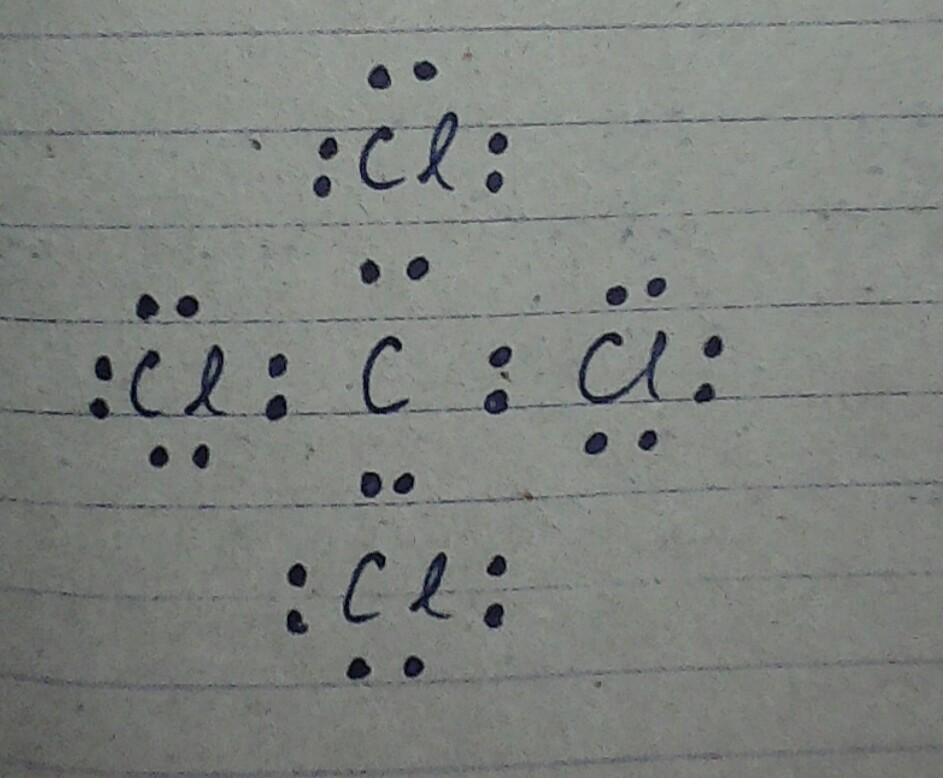Valance electrons: 32

Bond pairs = 4

Lone pairs = 12

Sigma bonds = 4

Pi-bonds = 0

Electron Pair Geometry : Tetrahedral

Molecular shape : Tetrahedral

CCl4 is a non-polar molecule.

--------------------

Molecule CHCl3 Lewis Structure: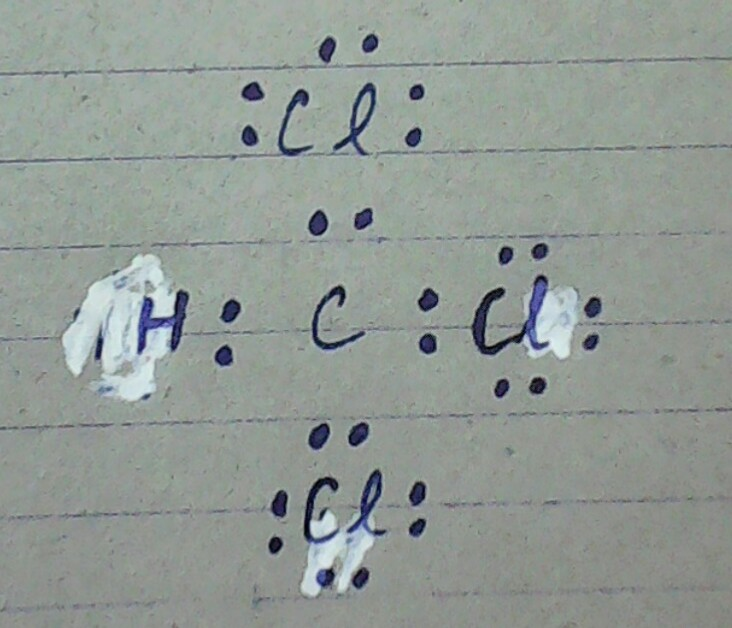Valance electrons: 26

Bond pairs : 4

Lone pairs : 9

Pi- bonds : 0

Electron pair Geometry : Tetrahedral

Molecular shape : Tetrahedral

CHCl3 is a polar molecule.

--------------------

#### Earn Coin

Coins can be redeemed for fabulous gifts.

Similar Homework Help Questions
• ### Name Section/CRN RESULTS (The choices of electron nair geometry and molecular shape are linear, bent, trigonal...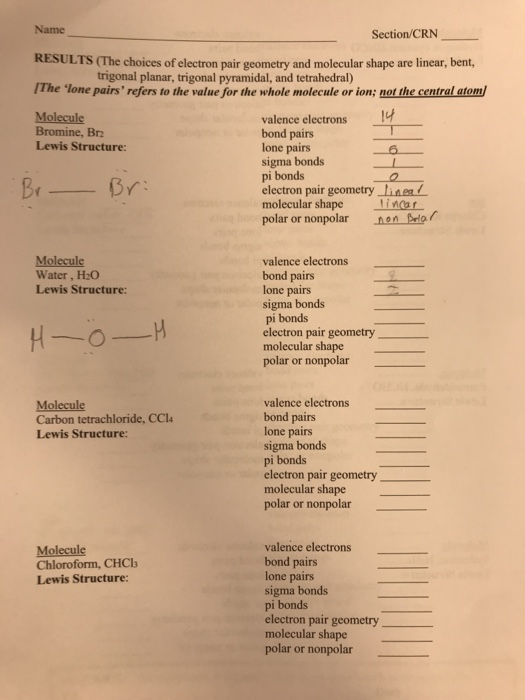Name Section/CRN RESULTS (The choices of electron nair geometry and molecular shape are linear, bent, trigonal planar, trigonal pyramidal, and tetrahedral) The 'lone pairs' refers to the value for the s' refers to the value for the whole molecule or ion; not the central atom Molecule Bromine, Br2 Lewis Structure: valence electrons bond pairs lone pairs sigma bonds pi bonds electron pair geometry Linea/ molecular shape linear polar or nonpolarn on Belor Bri Molecule Water, H20 Lewis Structure: valence electrons...

• ### valence electrons bond pairs lone pairs sigma bonds pi bonds electron pair geometry molecular shape polar...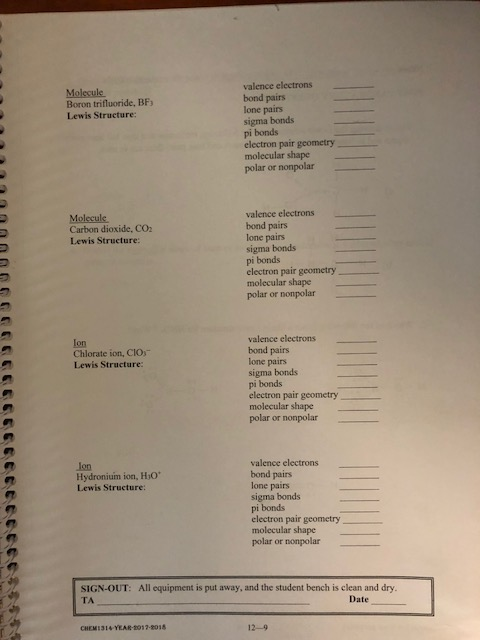valence electrons bond pairs lone pairs sigma bonds pi bonds electron pair geometry molecular shape polar or nonpolar Molecule Boron trifluoride, BFs Lewis Structure: valence electrons bond pairs lone pairs sigma bonds pi bonds electron pair geometry molecular shape polar or nonpolar Molecule Carbon dioxide, CO2 Lewis Structure valence electrons bond pairs lone pairs sigma bonds pi bonds electron pair geometry molecular shape polar or nonpolar lon Chlorate ion, CIOs Lewis Structure: valence electrons Ion Hydronium ion, HsO Lewis Structure:...

• ### Chemistry 2A Lab 11: Molecular Geometry Molecule or Ion Lewis Diagram Central Molecular Bond Atom Geometry Polari...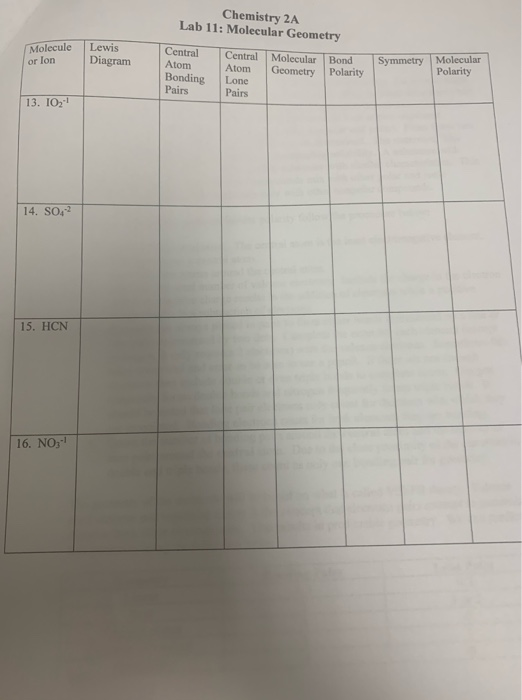Chemistry 2A Lab 11: Molecular Geometry Molecule or Ion Lewis Diagram Central Molecular Bond Atom Geometry Polarity Lone Pairs Symmetry Molecular Polarity Atom Bonding Pairs 13. IO2-1 14. SO42 15. HCN. 16. NO; To determine overall molecular polarity follow the procedure below: 1. Identify the central atom. The central atom is the least electronegative element. Hydrogen is not a central atom. 2. Place all other atoms around the central atom. 3. Calculate the total number of valence electrons. Include the...

• ### Complete the sentences to explain why CO2 and CCl4 are bothnonpolar even though they contain...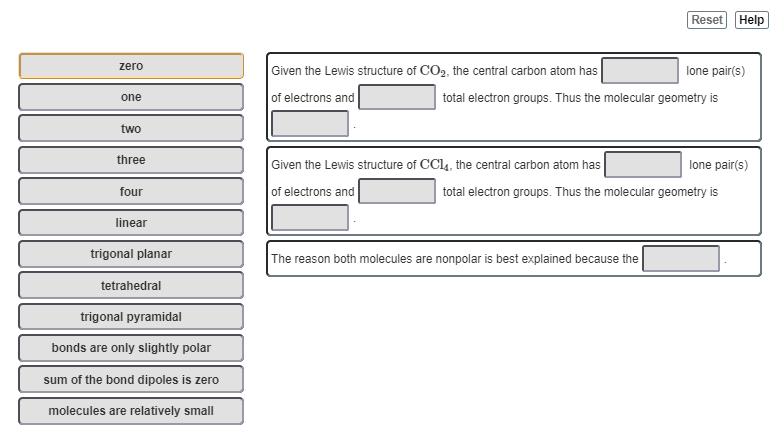Complete the sentences to explain why CO2 and CCl4 are both nonpolar even though they contain polar bonds.zero  one two three  four linear trigonal planar  tetrahedral trigonal pyramidal bonds are only slightly polar sum of the bond dipoles is zero molecules are relatively smallGiven the Lewis structure of CO2, the central carbon atom has lone pair(s) of electrons and total electron groups. Thus the molecular geometry is Given the Lewis structure of CCl4, the central carbon atom has lone pair(s) of electrons and...

• ### MODELS AND MOLECULAR GEOMETRY DATA QUESTION SHEET NAME Formula Lewis dot structure Shape: linear, bent (appropriate...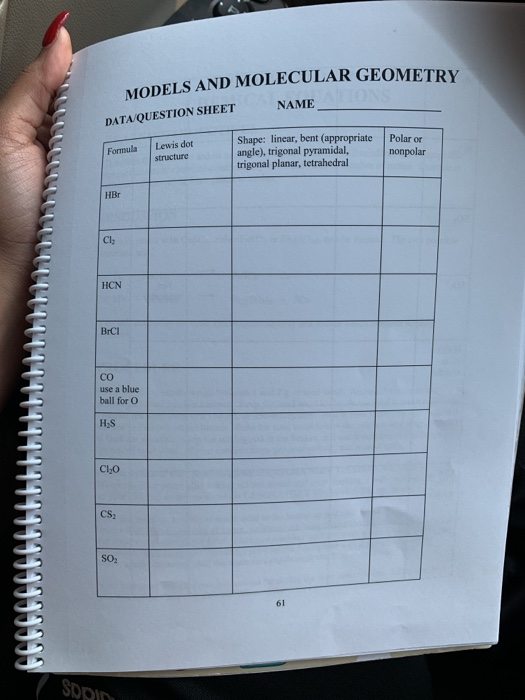MODELS AND MOLECULAR GEOMETRY DATA QUESTION SHEET NAME Formula Lewis dot structure Shape: linear, bent (appropriate angle), trigonal pyramidal, trigonal planar, tetrahedral Polar or nonpolar BrCI CO use a blue ball for o HS Cl, 666666666ԵԵԵԵԵԵԵԵԵԵՒՈՆ

• ### can you please decide wither it is covelant or ionic - Valence electrons -Electron pairs: -Molecular...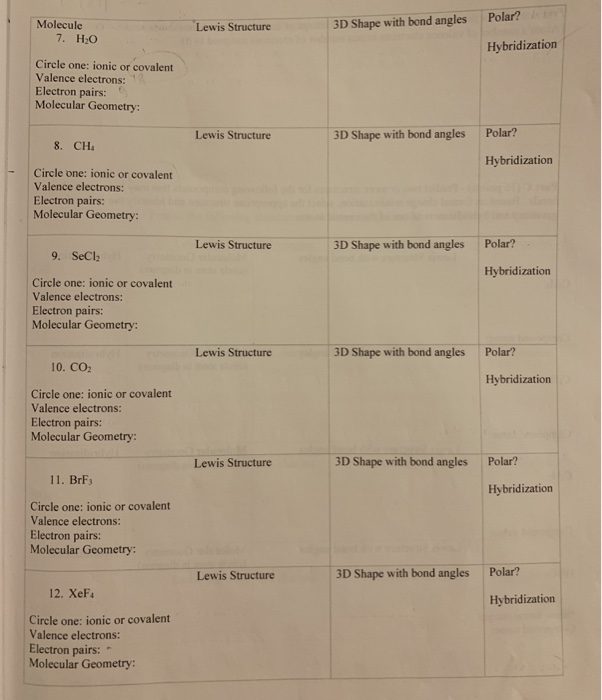can you please decide wither it is covelant or ionic - Valence electrons -Electron pairs: -Molecular Geometry and is it polar or not for each of the elements . Molecule 7. HO Polar? Lewis Structure 3D Shape with bond angles Hybridization Circle one: ionic or covalent Valence electrons: Electron pairs: Molecular Geometry: Lewis Structure 3D Shape with bond angles Polar? 8. CH: Hybridization Circle one: ionic or covalent Valence electrons: Electron pairs: Molecular Geometry: Lewis Structure 3D Shape with bond...

• ### Give the electron group geometry and molecular geometry for PC13 tetrahedral, bent trigonal planar, bent tetrahedral,...Give the electron group geometry and molecular geometry for PC13 tetrahedral, bent trigonal planar, bent tetrahedral, trigonal pyramidal trigonal planar, pyramidal trigonal pyramidal, see saw tetrahedral, T-shapted

• ### . Determine the electron geometry (eg), molecular geometry (mg), and polarity of PCl3. eg=tetrahedral, mg=trigonal pyramidal,...

. Determine the electron geometry (eg), molecular geometry (mg), and polarity of PCl3. eg=tetrahedral, mg=trigonal pyramidal, polar eg=tetrahedral, mg=bent, polar eg=linear, mg=linear, nonpolar eg=trigonal planar, mg=trigonal planar, nonpolar eg=trigonal pyramidal, mg=trigonal pyramidal, polar

• ### Draw the Lewis structure of XeCl. Include all lone pairs. Identify the molecular geometry of Xeci,...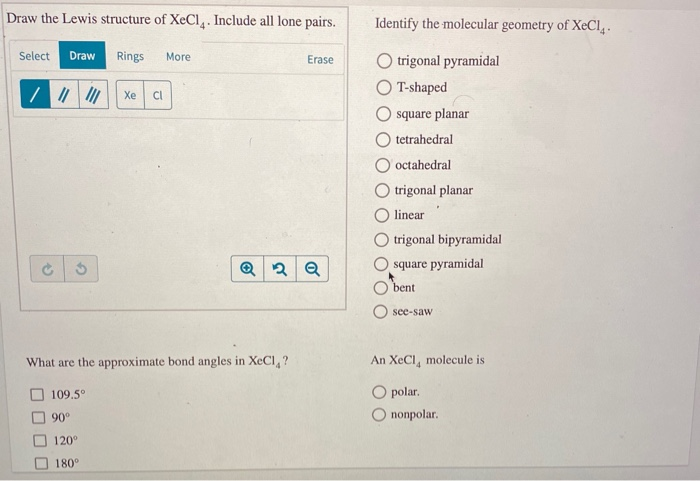Draw the Lewis structure of XeCl. Include all lone pairs. Identify the molecular geometry of Xeci, Select Draw Rings More Erase trigonal pyramidal T-shaped square planar O tetrahedral O octahedral trigonal planar linear trigonal bipyramidal square pyramidal bent O see-saw What are the approximate bond angles in XeCl,? An XeCl, molecule is O polar O nonpolar. 109.5° 90° 120° 180°

• ### Activity 2: Practicing VSEPR 3-SnCl FORMULA Total Valence ë FORMULA 2- BF 24 e 1-BeCl FORMULA:...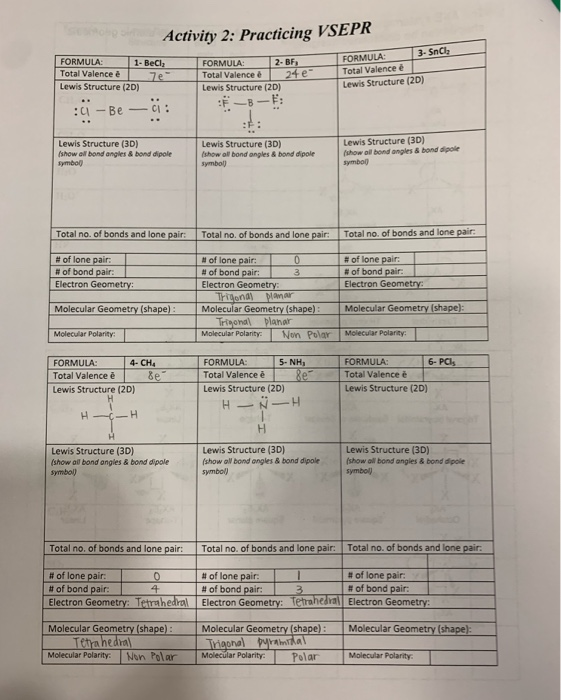Activity 2: Practicing VSEPR 3-SnCl FORMULA Total Valence ë FORMULA 2- BF 24 e 1-BeCl FORMULA: Total Valence e Total Valence è 7e Lewis Structure (2D) Lewis Structure (2D) Lewis Structure (2D) -Be CI Lewis Structure (3D) (show oll bond ongles & bond dipole symbol) Lewis Structure (3D) Lewis Structure (3D) (show oll bond angles & bond dipole (show oll bond angles & bond dipole symbol symbol) Total no. of bonds and lone pair Total no. of bonds and lone...